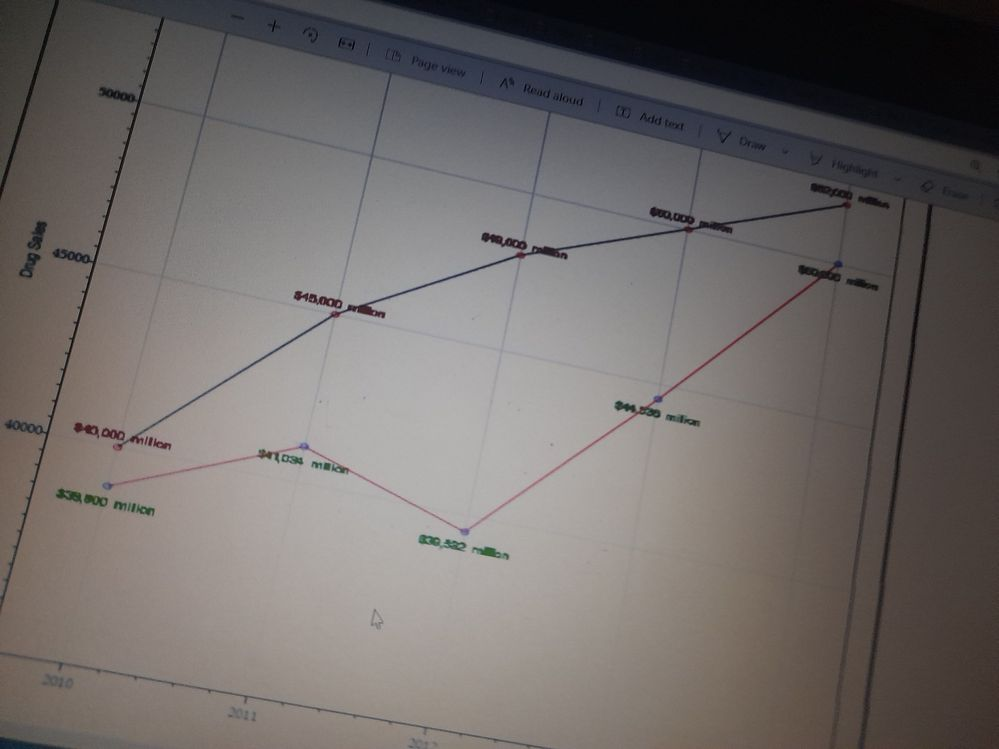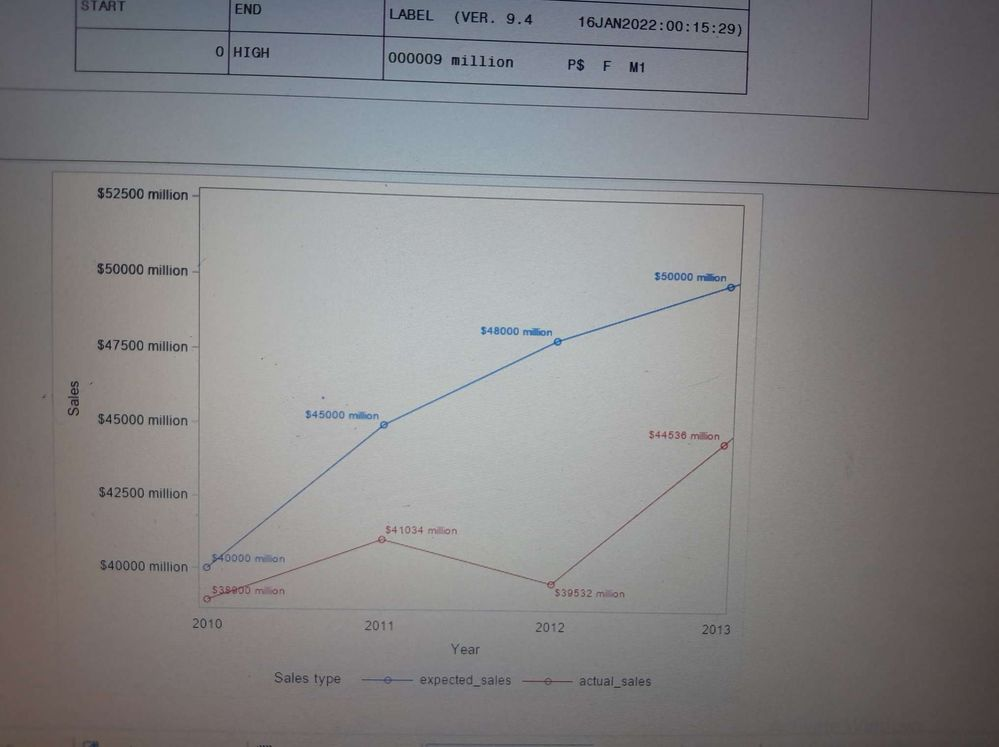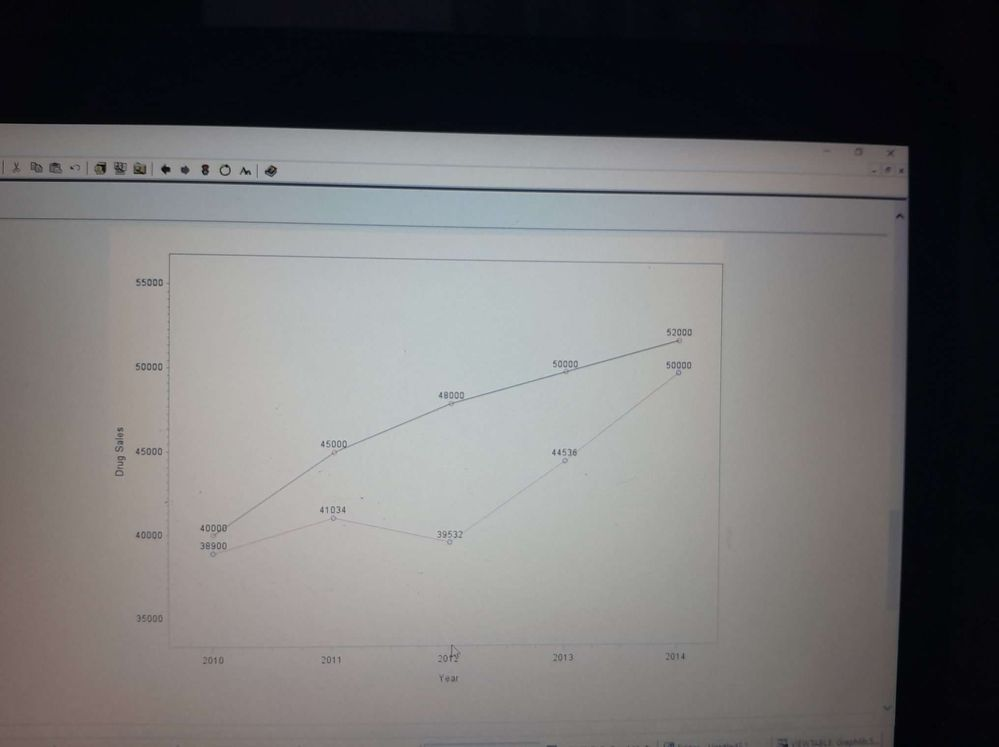## gplot annotation

Hi everyone!

I'm trying to create two different annotations for two line  plot.

data myanno;
set graphlib.sales_info;
length function \$10 text \$15;
retain xsys ysys "2" style "swiss" function "Label" size 1.5;
x=year;
y=put(expected_sales, dollar6.) || " million"; color="red"; text="sales1"; output;
y=put(actual_sales, dollar6.) || " million"; color="green"; text="sales2"; output;
run;

This is the plot;

proc gplot data=graphlib.sales_info;
plot expected_sales*year actual_sales*year/ overlay
vaxis=axis1 haxis=axis2
anno=myanno;
symbol1 v=circle cv=rose l=1 c=charcoal i=join w=1;
symbol2 v=circle cv=blue l=1 c=SALMON i=join w=1;
axis1 order=(35000,40000,45000,50000,55000)
label= (font="arial" angle=90"Drug Sales" )
minor=(n=10)
offset=(1,1)cm;
axis2 order=(2010,2011,2012,2013,2014)
label= (font="arial" "Year" )
minor=(n=3)
offset=(1.5,1.5)cm;
run;
quit;

LOG messag;

NOTE: ERROR DETECTED IN ANNOTATE= DATASET WORK.MYANNO.
USE THE YC VARIABLE FOR DATA VALUES WHEN TYPE IS CHARACTER
NOTE: ERROR LIMIT REACHED IN ANNOTATE PROCESS. PROCESSING IS TERMINATED.
NOTE: PROCESSING TERMINATED BY INDIVIDUAL ERROR COUNT.
NOTE: 1 TOTAL ERRORS.

Any clue how I can resolve this?

1 ACCEPTED SOLUTION

Accepted Solutions

## Re: gplot annotation

Is there a reason you are using Gplot instead of SGPLOT?

The error is pretty clear:

```LOG messag;

NOTE: ERROR DETECTED IN ANNOTATE= DATASET WORK.MYANNO.
USE THE YC VARIABLE FOR DATA VALUES WHEN TYPE IS CHARACTER```

Your annotate data is building a y variable with these lines:

```y=put(expected_sales, dollar6.) || " million"; color="red"; text="sales1"; output;
y=put(actual_sales, dollar6.) || " million"; color="green"; text="sales2"; output;```

Y in an annotate set must be numeric. It is very obviously not such.

I'm not so sure how useful having text like "sales1" and "sales2" scattered all over graph will actually be but maybe you have so few points it sort of makes sense.

Here is an example that I assume is somewhat similar to what you might be attempting that uses the newer SGPLOT and some of the options that reduce the need for an annotate data set. Note use of a small data set that you can run the code with.

```/* some data similar to yours*/
data example;
input year expected_sales actual_sales;
label expected_sales='Expected Sales'
actual_sales  ='Actual Sales';
datalines;
2010  10000 12500
2011  11000 12000
2012  11500 13000
2013  12000 11500
;

/* reshape to use the GROUP option in graphing*/
proc transpose data=example out=toplot;
by year;
var expected_sales actual_sales;
run;

/* custom format to display the values I think you
may have been attempting to show with the annotate
*/
proc format;
picture mymillions 0-high= '000009 million' (prefix='\$');
run;

proc sgplot data=toplot;
styleattrs datacontrastcolors=(blue red);
series x=year y=col1 /group=_label_ markers datalabel
;
label  year='Year'
col1 ='Sales'
_label_='Sales type'
;
format col1 mymillions.;
xaxis values=(2010 to 2013 by 1);
run;```

The Group = option in plots is a very powerful tool for creating multiple bars/lines/markers/text based on the value of the group. The defaults use your current ODS Style but you can override those with either  Styleattrs statement(s) as shown or a DATTRMAP auxiliary data set that displays colors, line types, marker symbols specified per value of the group variable.

So I reshape data similar to what I think yours is to create variables to use for Group, default from Proc Transpose either _label_ , if the value variables have lables (note that I added such because it creates nicer text easily in the graph) or _name_ , the original name of a variable.

The custom format is more flexible than having to use put() and concatenates to get the desired text.

The Sgplot and Sgpanel procedures will allow use of another variable than the actual plotted variable to provide the Datalabel , text for the points, and a format for that variable as well. Again reducing the need for annotate.

There are still annotate features for more complex needs but those usually are not quite tied to the values of the plotted variables.

If you would like something resembling working code for a solution you need to provide example data in the form of a data step. Instructions here: https://communities.sas.com/t5/SAS-Communities-Library/How-to-create-a-data-step-version-of-your-dat... will show how to turn an existing SAS data set into data step code that can be pasted into a forum code box using the </> icon or attached as text to show exactly what you have and that we can test code against.

10 REPLIES 10

## Re: gplot annotation## Re: gplot annotation

Is there a reason you are using Gplot instead of SGPLOT?

The error is pretty clear:

```LOG messag;

NOTE: ERROR DETECTED IN ANNOTATE= DATASET WORK.MYANNO.
USE THE YC VARIABLE FOR DATA VALUES WHEN TYPE IS CHARACTER```

Your annotate data is building a y variable with these lines:

```y=put(expected_sales, dollar6.) || " million"; color="red"; text="sales1"; output;
y=put(actual_sales, dollar6.) || " million"; color="green"; text="sales2"; output;```

Y in an annotate set must be numeric. It is very obviously not such.

I'm not so sure how useful having text like "sales1" and "sales2" scattered all over graph will actually be but maybe you have so few points it sort of makes sense.

Here is an example that I assume is somewhat similar to what you might be attempting that uses the newer SGPLOT and some of the options that reduce the need for an annotate data set. Note use of a small data set that you can run the code with.

```/* some data similar to yours*/
data example;
input year expected_sales actual_sales;
label expected_sales='Expected Sales'
actual_sales  ='Actual Sales';
datalines;
2010  10000 12500
2011  11000 12000
2012  11500 13000
2013  12000 11500
;

/* reshape to use the GROUP option in graphing*/
proc transpose data=example out=toplot;
by year;
var expected_sales actual_sales;
run;

/* custom format to display the values I think you
may have been attempting to show with the annotate
*/
proc format;
picture mymillions 0-high= '000009 million' (prefix='\$');
run;

proc sgplot data=toplot;
styleattrs datacontrastcolors=(blue red);
series x=year y=col1 /group=_label_ markers datalabel
;
label  year='Year'
col1 ='Sales'
_label_='Sales type'
;
format col1 mymillions.;
xaxis values=(2010 to 2013 by 1);
run;```

The Group = option in plots is a very powerful tool for creating multiple bars/lines/markers/text based on the value of the group. The defaults use your current ODS Style but you can override those with either  Styleattrs statement(s) as shown or a DATTRMAP auxiliary data set that displays colors, line types, marker symbols specified per value of the group variable.

So I reshape data similar to what I think yours is to create variables to use for Group, default from Proc Transpose either _label_ , if the value variables have lables (note that I added such because it creates nicer text easily in the graph) or _name_ , the original name of a variable.

The custom format is more flexible than having to use put() and concatenates to get the desired text.

The Sgplot and Sgpanel procedures will allow use of another variable than the actual plotted variable to provide the Datalabel , text for the points, and a format for that variable as well. Again reducing the need for annotate.

There are still annotate features for more complex needs but those usually are not quite tied to the values of the plotted variables.

If you would like something resembling working code for a solution you need to provide example data in the form of a data step. Instructions here: https://communities.sas.com/t5/SAS-Communities-Library/How-to-create-a-data-step-version-of-your-dat... will show how to turn an existing SAS data set into data step code that can be pasted into a forum code box using the </> icon or attached as text to show exactly what you have and that we can test code against.

## Re: gplot annotation

Okay, thank you. Not clear at the moment, but I will go through the recommended material and try to implement it on my code.

## Re: gplot annotation

Okay, thank you. Not clear at the moment, but I will go through the recommended material and try to implement it on my code.

The Sgplot and Sgpanel provide a great many more types of graphs, generally easier to overlay them, though there are restrictions involving combinations of graphs that do summarys, like bars, and continuous values like scatter and series plots.

Several of the ideas from Gplot are there but moved to different places, such as Keylegend statement to control some appearance of the legends instead of the Legend statements. Most things are quite a bit easier after you get over the transition.  I say that having learned Gplot with pen plotters in SAS 5.

## Re: gplot annotation

I was able to implement your solution, and it came out as expected. Though I would have preferred if the y-axis value contained no dollar sign (4000 million instead of \$4000 million), I will also need to manipulate the colors of the lines, and enlarge the frameThis is how it came out. My major challenge, though, is that I do not understand the logic behind graphs; hence, I can only do little manipulation. Most of the books I have contain little on graphs. Can you recommend any text that can help me grasp graphs easily?

Thank you very much, sir.

## Re: gplot annotation

The dataset;

data WORK.SALES_INFO;
infile datalines dsd truncover;
input year:32. expected_sales:32. actual_sales:32.;
datalines;
2010 40000 38900
2011 45000 41034
2012 48000 39532
2013 50000 44536
2014 52000 50000
;
run;Ksharp
Super User

## Re: gplot annotation

Calling @GraphGuy

## Re: gplot annotation

Rather than annotating the labels for each value on the lines, it would probably be easier to use the pointlabel feature of the symbol statement.

First, add a variable to the dataset containing the text you want to use as the label, such as ...

expected_sales_label=put(expected_sales, dollar6.) || " million";

And then specify that as the pointlabel in the appropriate label statement, something like ...

symbol1 v=circle cv=rose l=1 c=charcoal i=join w=1 pointlabel=("#expected_sales_label");

## Re: gplot annotation

data graphlib.myanno;
set graphlib.sales_info;
length function expected_sales_label actual_sales_label \$20;
retain xsys ysys "2" style "swiss" when "a" function "label";
color="red"; size=1;
x=year;
expected_sales_label=put(expected_sales, dollar6.) || " million";
actual_sales_label=put(actual_sales, dollar6.) || " million";
run;

proc gplot data=graphlib.sales_info;
plot expected_sales*year actual_sales*year/ overlay
vaxis=axis1 haxis=axis2
anno=graphlib.myanno;
symbol1 v=circle cv=rose l=1 c=charcoal i=join w=1 pointlabel=("#expected_sales_label");
symbol2 v=circle cv=blue l=1 c=SALMON i=join w=1 pointlabel=("#actual_sales_label");;
axis1 order=(35000,40000,45000,50000,55000)
label= (font="arial" angle=90"Drug Sales" )
minor=(n=10)
offset=(1,1)cm;
axis2order=(2010,2011,2012,2013,2014)
label= (font="arial" "Year" )
minor=(n=3)
offset=(1.5,1.5)cm;
run;
quit;

How can I manipulate the color of the labels, add the dollar prefix and the marker at the bottom of the graph with gplot?

## Re: gplot annotation

The symbol statement's pointlabel= option allows you to specify several characteristics of the text. Have a look at the symbol statement's documentation! 🙂

Discussion stats
• 10 replies
• 469 views
• 2 likes
• 4 in conversation